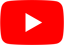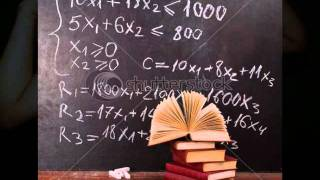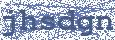Math Suks
Jimmy Buffett

## JIMMY BUFFETT

Math Suks Lyrics

If necessity was the mother of invention
Then I'd like to kill the guy who invented this
The numbers come together in some kind of third dimension
A regular algebraic bliss

Let's starts with something simple
Like 1 and 1 ain't 3
Any 2 plus 2 will never get you 5
There's fractions in my subtraction
And x don't equal y
But my homework is bound to multiply

Math suks (math suks), math suks (math suks)
I'd like to burn this text book
I hate that stuff so much
Math suks (math suks), math suks (math suks)
Sometimes I think that I don't know that much
But math suks

I got so bored with my homework
I turned on the tv
The beauty pageant winners were all smiling through their teeth
And they asked the new miss america
Hey babe can you add up all those bucks
She looked puzzled then just said "math suks"

Math suks (math suks), math suks (math suks)
You don't even have to spell it
All you have to do is yell it
Math suks (math suks), math suks (math suks)
Sometime times I think that I don't know that much
But math suks

Geometry and trigonometry and if they don't tax your brain
There are numbers to big to be named
Numerical precision is a science with a mission
And I think its' gonna drive me insane

Parents fighting with their children
And the congress can't agree
Teachers and their students are all jousting constantly
Management and labor keep rattling old sabres
Quacking like those peabody ducks

Math suks (quack quack), math suks (quack quack)
You don't even have to spell it
All you have to do is yell it
Math suks, that's right, math suks, you got it
Sometime times I think that I don't know that much
But math suks
All right there goes our g rating

Math suks, math suks, math suks the big one
Math suks, math suks, math suks the big one
Math suks, math suks, math suks the big one
Math suks, math suks, math suks, math suksHottest Lyrics with Videos
9bf2dac6a5cb18c2b85b94b317c157df